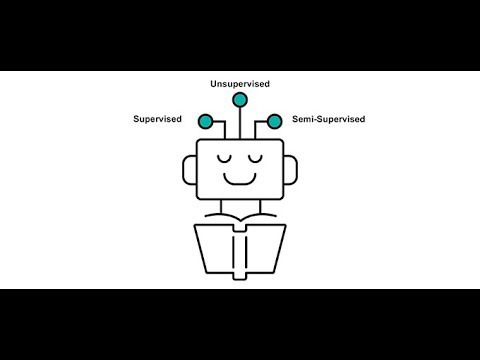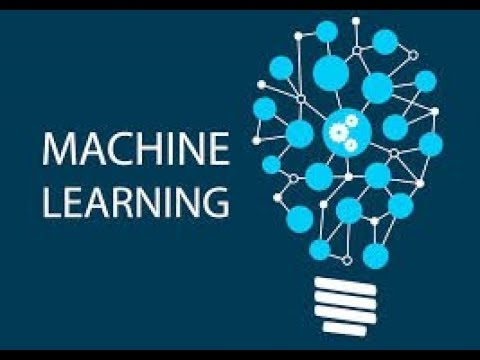Select Page

45,575.00

Course List

• PYTHON FOR ANALYTICS & MACHINE LEARNING
• MACHINE LEARNING WITH PYTHON
• DEEP LEARNING AND ARTIFICIAL INTELLIGENCE
 Select Date Choose an option12 Oct 201907 Dec 2019Clear
SKU: N/A Category:

Machine Learning & Artificial Intelligence with Python

The number of data science professionals in US alone would be around 700,000 by 2020. For aspiring Data Scientists Python is the most important language to learn because of its rich ecosystem. Python is among top 3 languages officially listed and used by Google. Python currently is used in more than 75% of all data analysis work across the world. Python programmers requirement including Machine Learning knowledge is expected to reach approximately 400,000 by end of 2018

Preview VideosPYTHON FOR ANALYTICS & MACHINE LEARNING

• Fundamentals of Python
• Numpy Arrays
• Introduction to Matrices
• Pandas DataFrames
• Importing data into DataFrames
• Visualization
• Introduction to Matplotlib

MACHINE LEARNING WITH PYTHON

Introduction of Data Science and Machine Leaning
Introduction Python

Statistics for Machine Learning
• Inferential Statistics
• Descriptive Statistics

Theory of Distribution
• Probability Distribution
• Sampling Data
• Types of Sampling

Regression & Modelling
• Simple Linear Regression
• Multiple Linear Regression
• Polynomial Regression
• Decision Tree Regression
• Random Forest Regression
• Logistic Regression

K-Nearest Neighbors (K-NN)
Support Vector Machine (SVM)
Naive Bayes
Decision Tree Classification
Random Forest Classification
• K-Means , Hierarchical Clustering

DEEP LEARNING AND ARTIFICIAL INTELLIGENCE

• Apriori
• Upper Confidence Bound (UCB)
• Thompson Sampling
• Natural Language Processing
• Artificial Neural Networks